Mobile QR Code1. (D. Kim and D. Im are with the Division of Electronics Engineering, Chonbuk National University, Jollabuk-do 561-756, Korea.)
2. (I.-Y. Lee is with the Department of Electronic Engineering, Chosun University, Gwangju 61452, Korea. )

CMOS, negative resistance, on-chip spiral inductor, passive element, PTAT current source, PVT, quality factor, singled-ended

## I. INTRODUCTION

The on-chip spiral inductors fabricated on CMOS substrate suffers from poor quality factor ($Q$-factor) due to excessive resistance and substrate losses. Several layout and semiconductor process techniques such as patterned ground shield, high resistivity silicon substrate, differential excitation technique, n-well formation, the formation of porous silicon, and microelectromechanical (MEMS) fabrication have been devised to enhance poor $Q$-factor of the on-chip spiral inductors, but the degree of improvement was small and most of the attempted process techniques were not compatible with a low cost standard CMOS technology. On the other hand, concerning the circuit techniques, the negative resistance (NR) circuit occupying very small silicon area has been widely used to improve $Q$-factor of lossy on-chip inductors in designing RF circuits such as amplifiers, filters, and oscillators. Especially, by adopting it to filter and amplifier circuits, the insertion loss, out-of-band (OOB) rejection and voltage gain performances were greatly enhanced at the cost of a little bit of noise and linearity performances-.

Fig. 1. Conventional negative resistance (NR) circuit in parallel with $LC$ tuned load of the LNA in.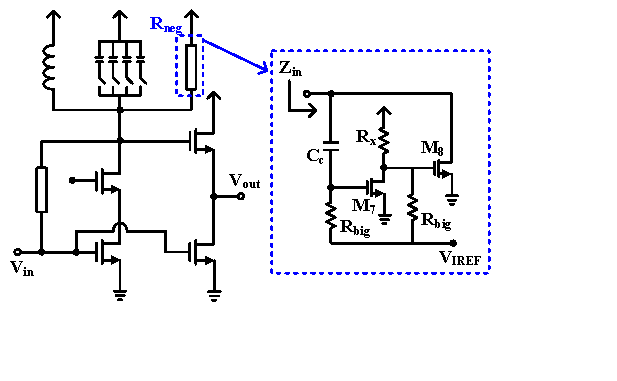In designing NR circuits for filter and amplifier circuit applications, it is very important to keep constant value of the negative resistance over process, voltage, and temperature (PVT) variations because those circuits are susceptible to oscillation and the effect of improving the $Q$-factor can be halved if the designed negative resistance deviates from the desired range. In this paper, we propose a PVT compensated NR circuit for high frequency applications that can improve the $Q$-factor of on-chip spiral inductors and ensure stability against all corner variations as well. In Section II, we present the proposed PVT compensated NR circuit and its small signal analysis.

Section III reports the simulation results of the designed NR circuit to verify the robustness over PVT variations, followed by the conclusion in Section IV.

## II. PVT COMPENSATED NR CIRCUIT DESIGN

Figure 1 shows the conventional negative resistance circuit reported in. The input impedance of the negative resistance cell consisting of $M_7$ and $M_8$ is given by $-1 /\left[g_{m 7} g_{m \delta}\left(R_{x} \| r_{o 7}\right)\right]$, where $g_{m7}$ and $g_{m8}$ are the trans-conductance values of $M_7$ and $M_8$ and $r_{o7}$ is the output resistance of $M_7$. By employing a negative resistance cell in parallel with the $LC$ tuned load, the effective parallel load resistance at the resonant frequency can be increased. This improves the voltage gain and OOB rejection characteristics of the LNA. Unfortunately, however, the negative resistance value varies greatly with PVT variations since there is no PVT compensation for the trans-conductance of $M_7$ and $M_8$ and the resistance of $R_x$. This makes the circuit more susceptible to oscillation when the designed negative resistance deviates from the target range.

Figure 2 shows the proposed PVT compensated NR circuit. In order to achieve a PVT-robust loop gain within the positive feedback loop, a common source (CS) amplifier with a diode-connected load whose voltage gain is determined by the transconductance ratio of the two NMOS transistors $M_1$ and $M_2$ is employed in the first stage. The input impedance ($Z_{in}$) and the real part ($R_{neg}$) of the proposed NR circuit are given as (1) and (2), where $g_{mn(n=1,2,3)}$, $r_{on(n=1,2,3)}$, and $C_{gdn(n=1,2,3)}$ are the trans-conductance, output resistance, and gate-to-drain capacitance of the transistors $M_{n(n=1,2,3)}$, $C_x$ and $C_y$ are the total parasitic capacitance at node $X$ and $Y$, and $R_y$ is the parallel impedance of $r_{o1}$, $r_{o2}$, and $1/g_{m2}$. At low frequencies near dc, assuming that $r_{o1}$ and $r_{o2}$ is much greater than $1/g_{m2}$, the negative resistance ($R_{neg}$) of (2) is simplified to -$g_{m2}$/($g_{m1}$$g_{m3}). Since the value is determined by the trans-conductance ratio, the proposed NR circuit exhibits a stable negative resistance value over PVT variation. However, unfortunately, the channel length modulation, one of the dominant short-channel effects, causes a reduction of output resistance (r_{o}) of the FET device in the adopted 130-nm process technology. Moreover, the reduction in r_{o} becomes even more severe when FET devices draw large currents to achieve low noise and high linearity performances. Therefore, it is desirable to make almost constant g_m over process and temperature variations in order to obtain a more stable negative resistance value. The adaptive body biasing-based threshold voltage (V_{th}) compensation circuit, which is composed of transistor M_4 and op-amp OP_1, was adopted to the proposed NR circuit in order to compensate process variation at a certain temperature. The proportional to absolute temperature (PTAT) current source employing a digitally trimmed resistor provides a constant reference current I_{REF} with diode-connected FET M_4 at a certain temperature, and the feedback operation of OP_1 makes constant gate-to-source voltage and V_{th} of M_4 over process variation by adjusting its body bias voltage. This ensures almost constant g_m of M_4 over process variation. In the same way the transistors M_1 and M_3 of the NR circuit core show nearly constant g_m over process variation by copying the reference current I_{REF} and sharing the automatically controlled body bias voltage of M_4. On the other hand, the PTAT current source was employed to compensate temperature variation. It greatly reduces the fluctuation of the negative resistance of the proposed NR circuit over temperature variation. In conclusion, a variety of circuit techniques including a CS amplifier with a diode-connected load, an adaptive body biasing-based V_{th} compensation circuit, and a PTAT current source significantly reduce the variation of the negative resistance value under PVT variations and contribute to the stable operation of the building block. ##### (1) Z_{i n} \approx \frac{1+j \omega\left(C_{y}+C_{g d 1}+C_{g d 3}\right) R_{y}}{-g_{m 1} g_{m 3} R_{y}+j \omega\left[C_{x}+\left(C_{y}+C_{g d 1}+C_{g d 3}\right) R_{y} / r_{o 3}+\left(C_{g d 1}+C_{g d 3}\right)\left(1+R_{y} g_{m 1}+R_{y} g_{m 3}\right)\right]} \operatorname{Re}\left(Z_{i n}\right)=R_{n e g} \approx ##### (2) \frac{-g_{m 1} g_{m 3} R_{y}+\omega^{2} R_{y}\left(C_{y}+C_{g d 1}+C_{g d 3}\right)\left[C_{x}+\left(C_{y}+C_{g d 1}+C_{g d 3}\right) R_{y} / r_{o 3}+\left(C_{g d 1}+C_{g d 3}\right)\left(1+R_{y} g_{m 1}+R_{y} g_{m 3}\right)\right]}{\left(g_{m 1} g_{m B}\right)^{2}+\omega^{2}\left[C_{x}+\left(C_{y}+C_{g d 1}+C_{g d 3}\right) R_{y} / r_{o 3}+\left(C_{g d 1}+C_{g d 3}\right)\left(1+R_{y} g_{m 1}+R_{y} g_{m 3}\right)\right]^{2}} ## III. SIMULATION RESULTS The proposed NR circuit was designed using a 0.13-{μ}m CMOS process, and the power consumption including V_{th} compensation circuit and PTAT current source is 3.2 mW at a 1.2-V supply voltage. Figures 3 shows the one-port S-parameter testbench schematic for the verification of the proposed NR circuit. The on-chip spiral inductor with inductance of 4 nH and Q-factor of 6 at 1 GHz was loaded by conventional and proposed NR circuits, and its overall inductance and Q-factor at around 1 GHz were simulated according to the variations of process and temperature. For a fair comparison, the power consumptions of two NR circuits are identical. Fig. 3. (a) One-port S-parameter testbench schematic, (b) two-port S-parameter testbench schematic, and simulated (c) inductance and (d) Q-factor of the on-chip spiral inductor loaded by the conventional NR circuit of Fig. 1 over process and temperature variations. TT, FF, and SS stand for typical, fast, and slow model of NMOS and PMOS, respectively.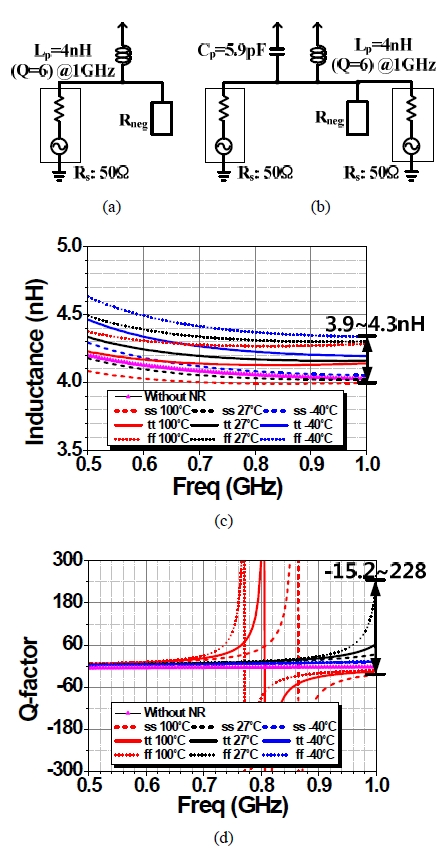Figures 3(c) and (d) show the simulated inductance and Q-factor of the on-chip spiral inductor loaded by conventional NR circuit of Fig. 1 over process and temperature variations. Because it is assumed that the circuit operates with an on-chip low-dropout regulator, the voltage variation is excluded in the simulation. The conventional NR circuit improves Q-factor of the on-chip spiral inductor by approximately 54 while keeping similar inductance of 4.2 nH at 1 GHz and typical condition (tt and 27^{\circ}C). However, the variation of Q-factor is very large over process and temperature (-40^{\circ}C ~ +100^{\circ}C) variations, which ranges from -15.2 to +228, and what is worse the circuit becomes unstable at a certain condition. Fig. 4. Simulated (a) inductance and (b) Q-factor of the on-chip spiral inductor loaded by the proposed NR circuit over process and temperature variations.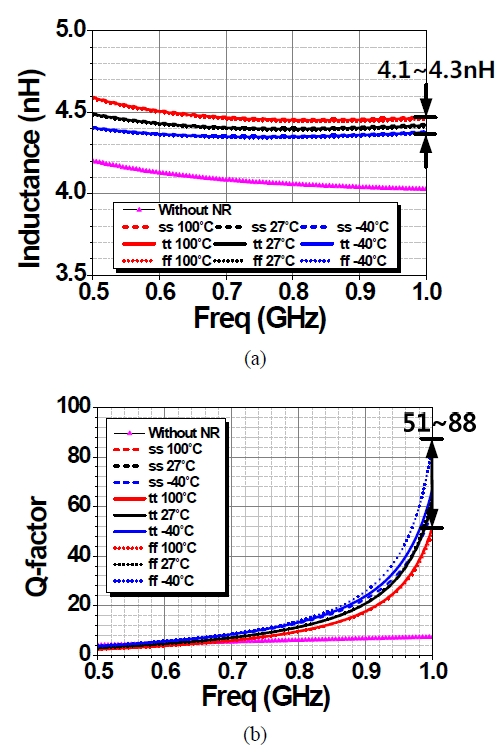Figures 4(a) and (b) present the simulated inductance and Q-factor of the on-chip spiral inductor loaded by the proposed NR circuit over process and temperature variations. The simulation condition is the same as that for Fig. 3. The improvement of Q-factor of the on-chip spiral inductor by the proposed NR circuit is similar to that by the conventional NR circuit at 1 GHz and typical condition, but the variation of Q-factor over process and temperature (-40^{\circ}C ~ +100^{\circ}C) variations is remarkably reduced, which ranges from +51 to +88. Due to the adopted various compensation techniques, the circuit is absolutely stable over all PVT variations. Fig. 5. Q-factor performance sensitivity of the on-chip spiral inductor loaded by (a) conventional and (b) proposed NR circuits, and the standard deviation of the negative resistance value of (c) conventional and (d) proposed NR circuits according to the process variation and mismatch effect of all transistors through Monte Carlo simulation with 100 runs.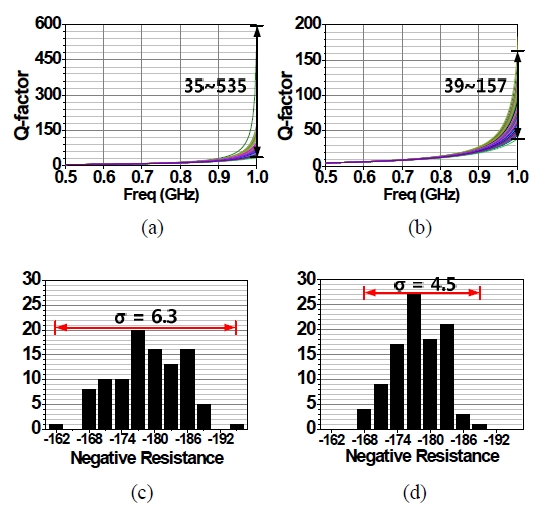Fig. 6. Simulated S_{21} and S_{11} of LC resonator loaded by (a) conventional and (b) proposed NR circuits using the testbench schematic of Fig. 3(b).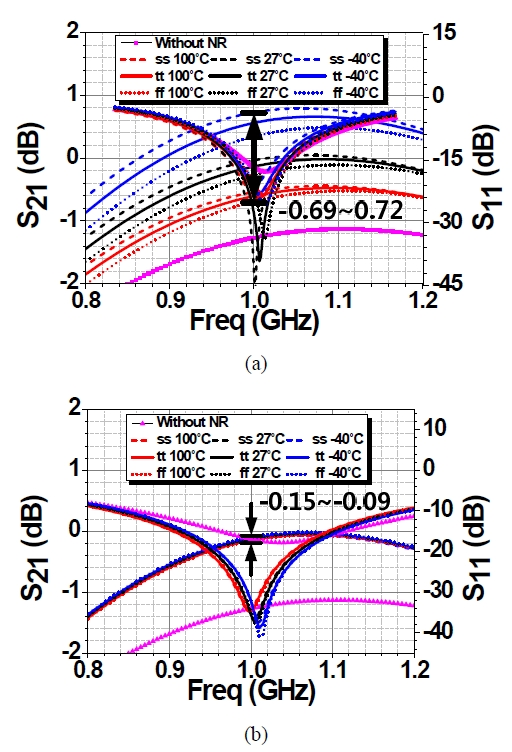Figures 5(a) and (b) show Q-factor performance sensitivity of the on-chip spiral inductor loaded by conventional and proposed NR circuits according to the process variation and mismatch effect of all transistors through Monte Carlo simulation with 100 runs. It can be seen that the Q-factor of the on-chip spiral inductor loaded by the proposed NR circuit is more stable than that by the conventional NR circuit. In Monte Carlo simulation, the standard deviation of negative resistance value of conventional and proposed NR circuits are 6.8 and 4.5, respectively, as shown in Figs. 5(c) and (d). Figures 6(a) and (b) show the simulated S$$_{21}$ and $S$$_{11} of LC resonator loaded by conventional and proposed NR circuits. The two-port S-parameter testbench schematic of Fig. 3(b) was used for the simulation. Because of the more stable negative resistance value over PVT variations, 1 GHz LC resonator loaded by the proposed NR circuit shows lower and more stable insertion loss (S$$_{21}$) than that loaded by the conventional NR circuit. The proposed NR circuit improves the insertion loss by at least 1 dB in comparison with $LC$ resonator without NR circuit.

Fig. 7. Simulated noise figure (NF) of $LC$ resonator loaded by (a) conventional and (b) proposed NR circuits using the testbench schematic of Fig. 3(b).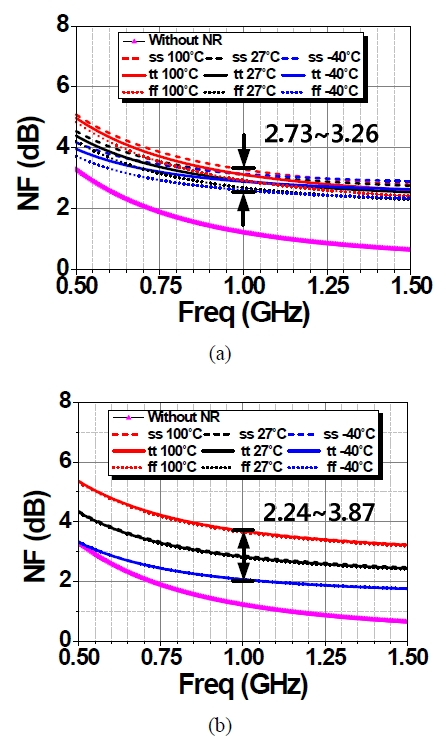Fig. 8. Simulated IIP3 performance of the proposed NR circuit.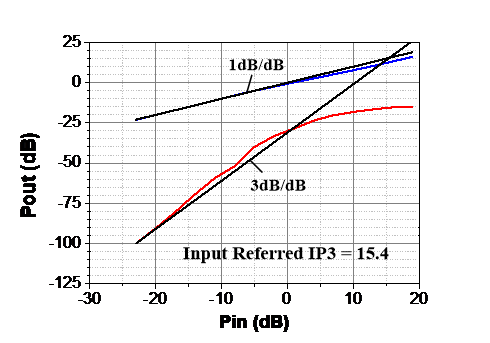One major drawback is the degradation of noise figure (NF) caused by the thermal noise generated from all transistors in the NR circuit. Figures 7(a) and (b) present the simulated NF of $LC$ resonator loaded by conventional and proposed NR circuits. The proposed NR circuit shows a slightly higher NF than the conventional one under all corner conditions because it is optimized to reduce the variation of the negative resistance value through various compensation techniques. In comparison with $LC$ resonator without NR circuit, the proposed NR circuit degrades the NF by about 2 dB at 1 GHz and typical condition. However, this will not be an issue because this increased NF can be alleviated by the gain of the block preceding it. Figure 8 shows the simulated input-referred third-order intercept point (IIP3) under typical corner conditions (tt, 1.2V, 27 ℃) when the proposed NR circuit is loaded with the two-port parallel $LC$ resonator shown in Fig. 3(b). Two tones at 1 GHz and 1.1 GHz are used. The simulated IIP3 is about +15.4 dBm, and this proves that the proposed NR circuit is quite linear.

## IV. CONCLUSIONS

The PVT compensated NR circuit was designed using a 0.13-${μ}$m CMOS process. The proposed NR circuit effectively suppresses the change of negative resistance caused by PVT variation only with negligible extra power consumption by applying various circuit technology such as a CS amplifier with a diode connection load, an adaptive body-biasing based $V_{th}$ compensation circuit and a PTAT current source. This allows the building blocks employing the proposed NR circuit to operate much more stable against PVT changes without significant power consumption.

## ACKNOWLEDGMENTS

This work was supported by Basic Science Research Program through the National Research Foundation of Korea (NRF) funded by the Ministry of Education (NRF-2018R1A2B6008816) and by the Research Base Construction Fund Support Program by Chonbuk National University in 2018. The CAD tool was supported by IDEC.

## REFERENCES

1
Yue C. P., Wong S. S., 12-14 June 1997, On-chip spiral inductors with patterned ground shields for Si-based RF IC’s, Symp. On VLSI Circuits Dig., pp. 85-592
Ashby K. B., Koullias I. A., Finley W. C., Bastek J. J., Moinian S., Jan 1996, High-Q inductors for wireless applications in a complementary silicon bipolar process, IEEE J. Solid-State Circuits, Vol. 31, pp. 4-93
Danesh M., Long J. R., Hadaway R. A., Harame D. L., 7-12 June 1998, A Q-factor engancement technique for MMIC inductors, IEEE MTT-S Int. Micrewave Symp. Dig., Vol. 1, pp. 183-1864
Kihong K., Kenneth O., Sept 1997, Characteristics of an intefrated spiral inductor with an underlying n-well, IEEE Trans. Electorn Devices, Vol. 44, pp. 1565-15675
Choong-Mo N., Young-Se K., Aug 1997, High-performance planar inductor on thick oxidized porous silicon (OPS) substrate, IEEE Microwave Guided Wave Lett., Vol. 7, No. 99, pp. 236-2386
Lu L.-H., Ponchak G. E., Bhattacharya P., Linda P., Katehi B., 2000, High-Q X-band and K-band Micromachined Spiral Inductors for Use in Si-based Integrated Circuits, in Dig. Topical Meeting on Silicon Monolithic Integrated Circuits in RF Systems, pp. 180-1127
Beare R., Plett C., Rogers J., 2012, Highly reconfigurable single-ended low noise amplifier for software defined radio applications, Proc. IEEE NEWCAS’12, pp. 549-5528
Wu Y., Ismail M., Olsson H., A novel CMOS fully differential inductorless RF bandpass filter, in Proc. IEEE Int. Symp. Circuits Syst.2000, pp. 149-1529
Strandvik Erlend, 2015, Compensation of Threshold Voltage for Process and Temperature Variations in 28nm UTBB FDSOI, M.S. dissertation, Department of Electronics and Telecommuniations, Norwegian University of Science and Technology